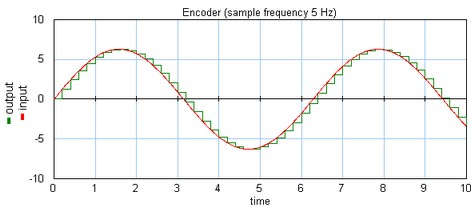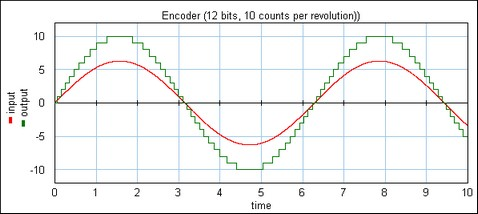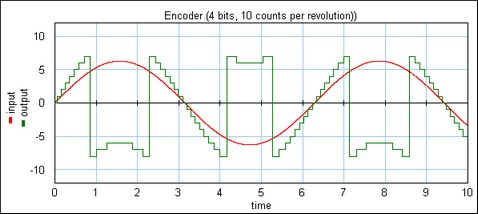﻿ 20-sim webhelp > Library > Iconic Diagrams > Mechanical > Rotation > Sensors > encoder

# encoder

## Library

Iconic Diagrams\Mechanical\Rotation\Sensors

Ideal

Absolute

Incremental

## Use

Domains: Continuous/Discrete. Size: 1-D. Kind: Iconic Diagrams (Rotation) / Block Diagrams.

## Description - Ideal

This is a model of an optical encoder combined with sampling. The Encoder has no internal friction. The output signal is equal to the sampled shaft shaft angle (in radians).

### Example

The shaft angle is equal to a sine with amplitude 2*pi (one revolution forward and one back), the sampling rate is 5 Hz.## Interface - Ideal

 Ports Description p Rotation port Causality fixed torque out (The output torque is zero) Outputs Description output sampled shaft angle (rad)

## Description - Absolute

This is a model of an optical encoder combined with counting logic and analog to digital conversion. The encoder has no internal friction. Counting is absolute. The shaft angle is measured with an accuracy of a given number of counts per revolution.

### Example

An encoder has an accuracy of 10 counts per revolution. This means an input signal with value 6.283185307 gives an output of 10. This is illustrated in the figure below. The input is a sine with amplitude 2*pi (one revolution forward and one back).### Overflow

This model has no overflow.

## Interface - Absolute

 Ports Description p Rotation port Causality fixed torque out (The output torque is zero) Outputs Description output number of counts Parameters counts counts per revolution.

## Description - Incremental

This is a model of an optical encoder combined with counting logic and analog to digital conversion. The encoder has no internal friction. Counting is incremental. The shaft angle is measured with an accuracy of a given number of counts per revolution and a window defined by the number of bits.

### Example

An encoder has an accuracy of 2000 counts per revolution. This means an input signal with value 6.283185307 gives an output of 2000.

### Example

An encoder has an accuracy of 10 counts per revolution and a 5 bits counter. This means an input signal with value 6.283185307 gives an output of 10 and the output is clipping between +15 and -16. This is illustrated in the figure below. The input is a sine with amplitude 2*pi (one revolution forward and one back).Encoder output without overflow.

The next figure shows the same settings but only 4 bits are used. This means the output is clipping between +7 and -8.Encoder output with overflow.

### Overflow

The maximum and minimum number of counts are equal to 2(bits-1) -1 and -2(bits-1). If the number of counts passes 2(bits-1) -1, counting continues at -2(bits-1) and vice versa.

## Interface - Incremental

 Ports Description p Rotation port Causality fixed torque out (The output torque is zero) Outputs Description output number of counts Parameters counts bits counts per revolution. maximum counting interval is between 2(bits-1) -1 and -2(bits-1).

## Limitations

The output of this model is a discrete signal. 20-sim will automatically detect the existence of discrete models. Each chain of discrete models will be assigned a specific sampletime. You can set this sample time to any desired value in the Simulator (choose Properties, Simulation and Discrete System).